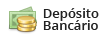# Ponteira Arrow Race Tech Kawasaki Ninja 400 18'~21'

Código: 71874 Marca:
Selecione a opção de Ponteira :
6x de R\$ 607,50
ou R\$ 3.353,40 via Depósito
Comprar Estoque: 60 dias úteis
6x de R\$ 607,50
ou R\$ 3.353,40 via Depósito
Comprar Estoque: 60 dias úteis
6x de R\$ 748,33
ou R\$ 4.130,80 via Depósito
Comprar Estoque: 60 dias úteis
6x de R\$ 670,83
ou R\$ 3.703,00 via Depósito
Comprar Estoque: 60 dias úteis
6x de R\$ 607,50
A partir de R\$ 3.645,00
ou R\$ 3.353,40 via Depósito
• 1x de R\$ 3.645,00 sem juros
• 2x de R\$ 1.822,50 sem juros
• 3x de R\$ 1.215,00 sem juros
• 4x de R\$ 911,25 sem juros
• 5x de R\$ 729,00 sem juros
• 6x de R\$ 607,50 sem juros
• 1x de R\$ 3.645,00 sem juros
• 2x de R\$ 1.822,50 sem juros
• 3x de R\$ 1.215,00 sem juros
• 4x de R\$ 980,36
• 5x de R\$ 795,67
• 6x de R\$ 672,63
• 7x de R\$ 584,82
• 8x de R\$ 519,03
• 9x de R\$ 467,92
• 10x de R\$ 427,08
• 11x de R\$ 393,72
• 12x de R\$ 365,96
• R\$ 3.353,40• 1x de R\$ 3.645,00 sem juros
• 2x de R\$ 1.822,50 sem juros
• 3x de R\$ 1.215,00 sem juros
• 4x de R\$ 911,25 sem juros
• 5x de R\$ 729,00 sem juros
• 6x de R\$ 607,50 sem juros
• 1x de R\$ 3.645,00 sem juros
• 2x de R\$ 1.822,50 sem juros
• 3x de R\$ 1.215,00 sem juros
• 4x de R\$ 980,36
• 5x de R\$ 795,67
• 6x de R\$ 672,63
• 7x de R\$ 584,82
• 8x de R\$ 519,03
• 9x de R\$ 467,92
• 10x de R\$ 427,08
• 11x de R\$ 393,72
• 12x de R\$ 365,96
• R\$ 3.353,40• 1x de R\$ 4.490,00 sem juros
• 2x de R\$ 2.245,00 sem juros
• 3x de R\$ 1.496,66 sem juros
• 4x de R\$ 1.122,50 sem juros
• 5x de R\$ 898,00 sem juros
• 6x de R\$ 748,33 sem juros
• 1x de R\$ 4.490,00 sem juros
• 2x de R\$ 2.245,00 sem juros
• 3x de R\$ 1.496,66 sem juros
• 4x de R\$ 1.207,64
• 5x de R\$ 980,13
• 6x de R\$ 828,56
• 7x de R\$ 720,40
• 8x de R\$ 639,35
• 9x de R\$ 576,39
• 10x de R\$ 526,09
• 11x de R\$ 484,99
• 12x de R\$ 450,80
• R\$ 4.130,80• 1x de R\$ 4.025,00 sem juros
• 2x de R\$ 2.012,50 sem juros
• 3x de R\$ 1.341,66 sem juros
• 4x de R\$ 1.006,25 sem juros
• 5x de R\$ 805,00 sem juros
• 6x de R\$ 670,83 sem juros
• 1x de R\$ 4.025,00 sem juros
• 2x de R\$ 2.012,50 sem juros
• 3x de R\$ 1.341,66 sem juros
• 4x de R\$ 1.082,57
• 5x de R\$ 878,62
• 6x de R\$ 742,75
• 7x de R\$ 645,79
• 8x de R\$ 573,14
• 9x de R\$ 516,70
• 10x de R\$ 471,61
• 11x de R\$ 434,77
• 12x de R\$ 404,11
• R\$ 3.703,00• 1x de R\$ 3.645,00 sem juros
• 2x de R\$ 1.822,50 sem juros
• 3x de R\$ 1.215,00 sem juros
• 4x de R\$ 911,25 sem juros
• 5x de R\$ 729,00 sem juros
• 6x de R\$ 607,50 sem juros
• 1x de R\$ 3.645,00 sem juros
• 2x de R\$ 1.822,50 sem juros
• 3x de R\$ 1.215,00 sem juros
• 4x de R\$ 980,36
• 5x de R\$ 795,67
• 6x de R\$ 672,63
• 7x de R\$ 584,82
• 8x de R\$ 519,03
• 9x de R\$ 467,92
• 10x de R\$ 427,08
• 11x de R\$ 393,72
• 12x de R\$ 365,96
• R\$ 3.353,40* Este prazo de entrega está considerando a disponibilidade do produto + prazo de entrega.